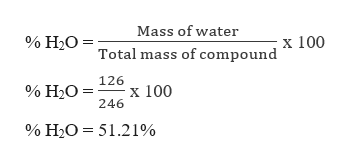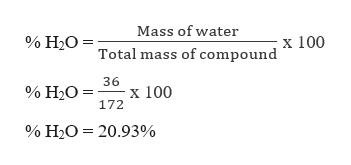# A. answer the following :  1. What are the formulas of the hydrated salts?Magnesium Sulfate______________________Calcium Sulfate___________________Copper(II) Sulfate__ 2. .What is the %H2O in each of these hydrated salts Magnesium Sulfate______________________Calcium Sulfate___________________Copper(II) Sulfate_________

Question
7 views

1. What are the formulas of the hydrated salts?
Magnesium Sulfate______________________
Calcium Sulfate___________________
Copper(II) Sulfate__

2. .What is the %H2O in each of these hydrated salts

Magnesium Sulfate______________________
Calcium Sulfate___________________
Copper(II) Sulfate_________
check_circle

Step 1

The formulae for the following hydrated salts are given as,

Step 2

Part A:

Magnesium sulfate heptahydrate (MgSO4.7H2O)

Mass of MgSO4.7H2O = (24 + 32 + 16 x 4) + (7 x 18)

Mass of MgSO4.7H2O = 246 u

Mass of water in MgSO4.7H2O = 7 x 18 = 126 uhelp_outlineImage TranscriptioncloseMass of water % H2O = x 100 Total mass of compound 126 x 100 246 % H20 = % H20= 51.21% fullscreen
Step 3

Part B:

Calcium Sulfate dihydrate (CaSO4.2H2O)

Mass of CaSO4.2H2O = (40 + 32 + 16 x 4) + 2 x 18

Mass of CaSO4.2H2O = 172 u

Mass of wa...help_outlineImage TranscriptioncloseMass of water % H2O= x 100 Total mass of compound 36 x 100 172 % H2O % H2O 20.93% fullscreen

### Want to see the full answer?

See Solution

#### Want to see this answer and more?

Solutions are written by subject experts who are available 24/7. Questions are typically answered within 1 hour.*

See Solution
*Response times may vary by subject and question.
Tagged in

### General Chemistry